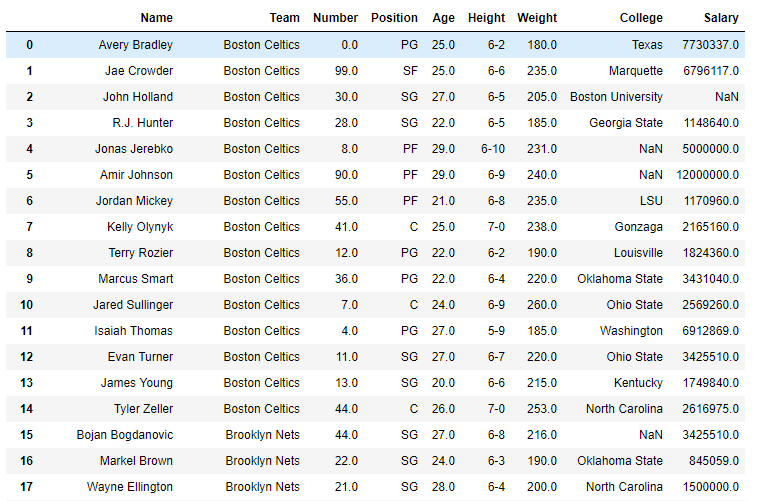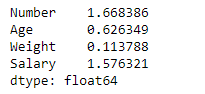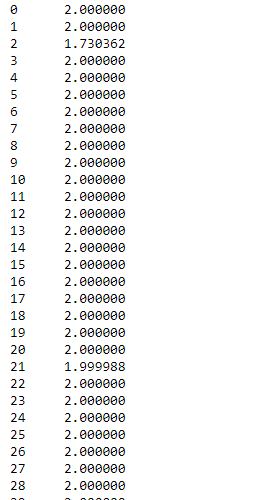GFG App
Open AppBrowser
Continue

# Python | Pandas dataframe.skew()

Python is a great language for doing data analysis, primarily because of the fantastic ecosystem of data-centric python packages. Pandas is one of those packages and makes importing and analyzing data much easier.

Pandas` dataframe.skew()` function return unbiased skew over requested axis Normalized by N-1. Skewness is a measure of the asymmetry of the probability distribution of a real-valued random variable about its mean. For more information on skewness, refer this link.

Pandas: DataFrame.skew(axis=None, skipna=None, level=None, numeric_only=None, **kwargs)

Parameters :
axis : {index (0), columns (1)}
skipna : Exclude NA/null values when computing the result.
level : If the axis is a MultiIndex (hierarchical), count along a particular level, collapsing into a Series
numeric_only : Include only float, int, boolean columns. If None, will attempt to use everything, then use only numeric data. Not implemented for Series.

Return : skew : Series or DataFrame (if level specified)

Example #1: Use `skew()` function to find the skewness in data over the index axis.

 `# importing pandas as pd ` `import` `pandas as pd ` ` `  `# Creating the dataframe  ` `df ``=` `pd.read_csv(``"nba.csv"``) ` ` `  `# Print the dataframe ` `df `Let’s use the `dataframe.skew()` function to find skewness

 `# skewness along the index axis ` `df.skew(axis ``=` `0``, skipna ``=` `True``) `

Output :Example #2: Use `skew()` function to find the skewness of the data over the column axis.

 `# importing pandas as pd ` `import` `pandas as pd ` ` `  `# Creating the dataframe  ` `df ``=` `pd.read_csv(``"nba.csv"``) ` ` `  `# skip the na values ` `# find skewness in each row ` `df.skew(axis ``=` `1``, skipna ``=` `True``) `

Output :My Personal Notes arrow_drop_up